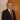# PROF. FIKRET ALIEV

## PROF. FIKRET ALIEV

Institute of Applied Mathematics, BSU, Azerbaijan

TITLE: Algorithm for solving the problem of optimal stabilization by output and their application

ABSTRACT: The paper is devoted to the solutions of high-accuracy of the synthesis problem of optimal regulators with the frequency-time method. The problem for finding the optimal regulators by output variable in the stationary and periodic cases is considered and an iteration scheme for solution such problems supported symbolic computation is proposed. The solutions of high-accuracy of the matrix algebraic equations of Riccati, Lyapunov and Sylvester are given. At first the inverse optimal stabilization problem by output variable is investigated. Using the method of linear matrix inequalities the computational algorithms are proposed. The obtained results have been applied to practice, including to the optimal control problem used in oil extraction of gas lift method.

SHORT BIOGRAPHY: Professor Fikret Aliyev is Doctor of Physical and Mathematical Sciences, Full member of Azerbaijan National Academy of Sciences and director of the Institute of Applied Mathematics of Baku State University.

He was awarded Doctor Degree for his work “Methods of solution to the problems of optimal control of dynamical systems”.

Main obtained results are: 1. The frequency method for solution the discrete problem of the synthesis of stationary systems which allows to solve the class of problems that is substantially broader than the class of problems solved by the standard algorithm of Kalmaya-Buci was developed. 2. The frequency relations for symmetric, stabilizing solutions of Riccati matrix algebraic equations were found, which play an important role in solution of a problem of finding optimal (stationary, non-stationary) regulators; 3. Based on a combination of frequency relations with the method of reducing matrices to the Schur form or with the projections generated by the eigenvectors of the Hamiltonian matrix whose corresponding eigenvalues lie in the right and left half-planes respectively the new formulas for stabilizing solutions of Riccati matrix algebraic equations were obtained.

Fikret Aliev is author of more than 200 publications and 7 monographs.

More Speakers